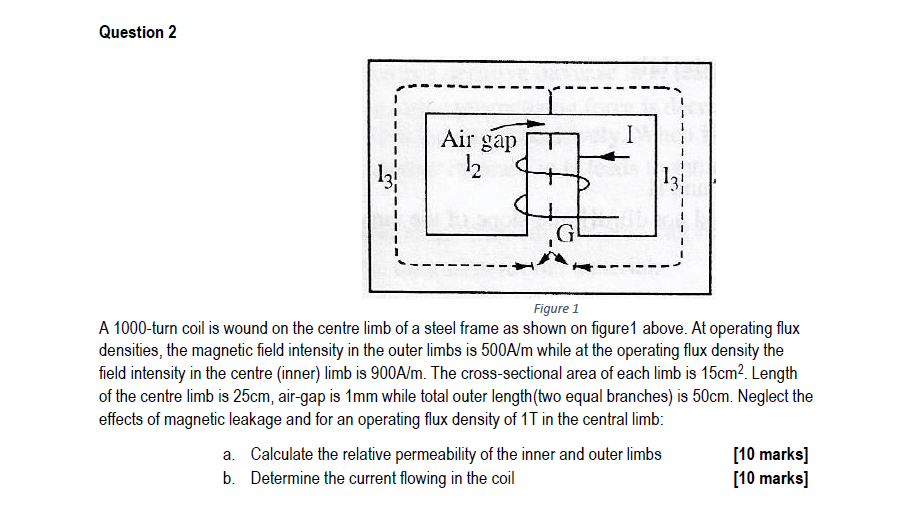# Question Question 2 Air gap I 12 Figure 1 A 1000-turn coil is wound on the centre limb of a steel frame as shown on figure1 above. At operating flux densities, the magnetic field intensity in the outer limbs is 500A/m while at the operating flux density the field intensity in the centre (inner) limb is 900A/m. The cross-sectional area of each limb is 15cmLength of the centre limb is 25cm, air-gap is 1mm while total outer length(two equal branches) is 50cm. Neglect the effects of magnetic leakage and for an operating flux density of 1T in the central limb: a. Calculate the relative permeability of the inner and outer limbs [10 marks] b. Determine the current flowing in the coil [10 marks]HZQJQH The Asker · Electrical EngineeringTranscribed Image Text: Question 2 Air gap I 12 Figure 1 A 1000-turn coil is wound on the centre limb of a steel frame as shown on figure1 above. At operating flux densities, the magnetic field intensity in the outer limbs is 500A/m while at the operating flux density the field intensity in the centre (inner) limb is 900A/m. The cross-sectional area of each limb is 15cmLength of the centre limb is 25cm, air-gap is 1mm while total outer length(two equal branches) is 50cm. Neglect the effects of magnetic leakage and for an operating flux density of 1T in the central limb: a. Calculate the relative permeability of the inner and outer limbs [10 marks] b. Determine the current flowing in the coil [10 marks]
More
Transcribed Image Text: Question 2 Air gap I 12 Figure 1 A 1000-turn coil is wound on the centre limb of a steel frame as shown on figure1 above. At operating flux densities, the magnetic field intensity in the outer limbs is 500A/m while at the operating flux density the field intensity in the centre (inner) limb is 900A/m. The cross-sectional area of each limb is 15cmLength of the centre limb is 25cm, air-gap is 1mm while total outer length(two equal branches) is 50cm. Neglect the effects of magnetic leakage and for an operating flux density of 1T in the central limb: a. Calculate the relative permeability of the inner and outer limbs [10 marks] b. Determine the current flowing in the coil [10 marks]